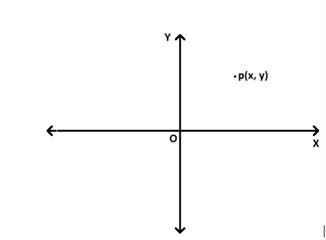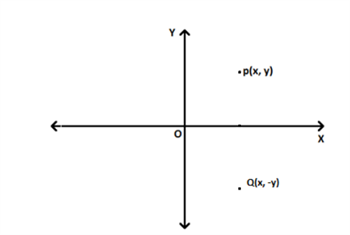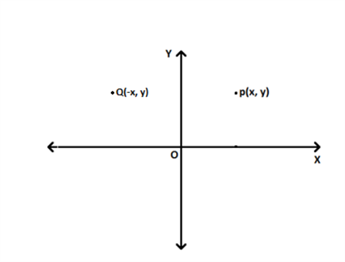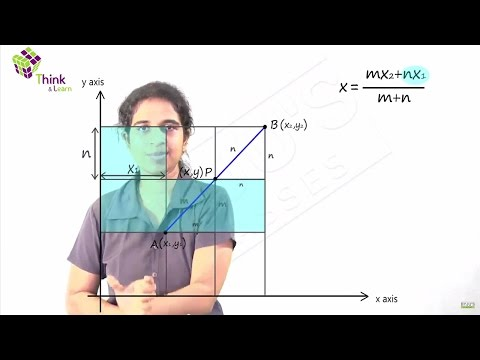# Two Dimensional Coordinate Geometry

Two-dimensional coordinate geometry deals about the coordinates which are represented in a coordinate plane. A coordinate plane has two axes, the one which is horizontal is known as $X-axis$ and the one which is vertical is known as $Y-axis$. A point $p(x,y)$ is represented in the $X-Y plane$ as shown below.$O$’ is known as origin whose coordinate is (0,0).

The perpendicular distance of $p(x,y)$ from $X-axis$ and $Y-axis$ is $’y'$ and $’x'$ respectively.

## Coordinate Geometry in Two Dimensional Plane

For example, the point $(2,3)$ is $3 ~units$ away from the $X-axis$ measured along the positive $Y-axis$ and $2~ units$ away from $Y-axis$ measured along the positive $X-axis$.

The points having $x$-coordinate as $‘0’$ lie on the $Y-axis$ and points having $y$-coordinate as $‘0’$ lie on the $X-axis$.

For example, the points $(2,0),(5,0)$ lie on $X-axis$ and the points $(0,-3),(0,7)$ lie on $Y-axis$.

Distance between two points:

Consider two points $A(x_1,y_1)$ and $B(x_2,y_2)$ in an $XY$ plane.

Then the distance between $A$ and $B$ is,

$AB$ = $√(x_2 ~-~ x_1)^2 + (y_2~-~y_1)^2$

For example; distance between the points $A(2,-3)$ and $B(5,1)$ is,

$AB$ = $√(5~-~2)^2 ~+~ (1-(-3))^2$ = $√3^2~ + ~4^2$ = $√25$ = $5~units$

Similarly, distance between a point $P(x,y)$ from the origin is,

$OP$ = $√(x-0)^2 ~+ ~(y-0)^2$ = $√(x^2~ +~ y^2)$

Reflection of a point across the X-axis

Reflection of a point $P(x,y)$ across the $X-axis$ is $Q(x,-y)$, which is found by changing the sign of the $y$-coordinate of $P(x,y)$.Reflection of a point across the Y-axis

Reflection of a point $P(x,y)$ across the $Y-axis$ is $Q(-x,y)$, which is found by changing the sign of the

$x$-coordinate of $P(x,y)$.Section Formula:

Consider two points $A(x_1,y_1)$ and $B(x_2,y_2)$ in an $XY$ plane.

$P(x,y)$ is a point which divides the line segment $AB$ internally in the ratio $m:n$,

Then, the $x$-coordinate of $P$ is,

$x$ = $\frac{m~x_2~ + ~n~x_1}{m~ + ~n}$

the $y$-coordinate of $P$ is,

$y$ = $\frac{m~y_2~ + ~n~y_1}{m~ + ~n}$

If $m$ = $n$, then $P$ is the mid-point of the line segment $AB$. Then coordinates of the point $P$ is,

$(\frac{x_1 ~+~ x_2}{2}, \frac{y_1 ~+ ~y_2}{2}$)

If the point $P(x,y)$ is dividing the line segment $AB$ externally in the ratio $m:n$,

Then, the $x$-coordinate of $P$ is,

$x$ = $\frac{m~x_2~ – ~n~x_1}{m ~- ~n}$

they-coordinate of $P$ is,

$y$ = $\frac{m~y_2 ~- ~n~y_1}{m~ – ~n}$

Example: Find the coordinates of the point which divides the line segment joining $P(-3,-4)$ and

$Q(6,8)$ in the ratio $1:2$.

Let $M(x,y)$ be the point which divides $PQ$ in the ratio $1:2$, then

$x$ = ${1 ~×~ 6 ~+~ 2~ ×~ (-3)}{1 ~+~ 2}$ = $\frac{6~ -~ 6}{3}$ = $0$

$y$ = $\frac{1 ~×~ 8 ~+~ 2~ ×~ (-4)}{1 ~+ ~2}$ = $\frac{8 – 8}{3}$ = $0$

Therefore origin $(0,0)$ divides the point $PQ$ in the ratio $1:2$.

Area of a triangle:

The area of the triangle whose vertices are $(x_1,y_1 ),(x_2,y_2)$ and $(x_3,y_3)$ is

$\frac{1}{2}|x_1 (y_2~ -~ y_3)~ + ~x_2(y_3~ – ~y_1)~ +~ x_3(y_1~ – ~y_2)|$

If the area of a triangle whose vertices are $(x_1,y_1),(x_2,y_2)$ and $(x_3,y_3)$ is zero, then the three points are collinear.We have learnt about two-dimensional coordinate geometry till now. To solve more problems on topic Coordinate Geometry visit BYJU’S which provides detailed and step by step solutions to all questions in an NCERT Books. Also, take free tests to practice for exams.